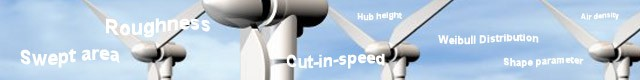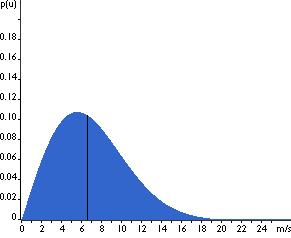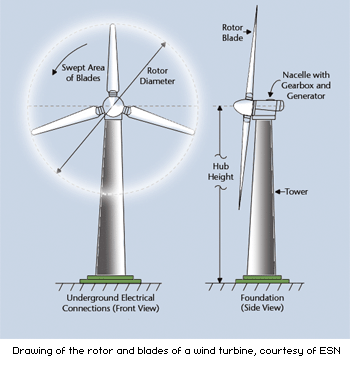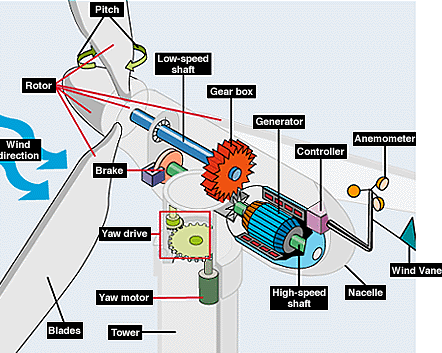# Wind Turbine Calculator## Energy and Cost Calculations – Wind Turbines

Important!

Using these energy calculators provides rough figures. You should use the results to get a rough estimate of the size and cost of your required system. To get more accurate information it is advised that you consult an accredited renewable energy supplier.

For information about how wind turbines work, see the Wind Power article this web site.

Also, be sure to stay on top of your country’s renewable energy subsidy programs as this will make a very big difference to the ultimate costs (see Subsidy Programs this web site).

Wind Turbine Calculators

The objective of using a wind turbine system calculator is to determine the size, cost and eventual cost savings of the wind turbine you will need based on your electricity requirements and access to wind. To best understand how these calculations are made it is advised to have a look at the ‘Terms’  section below.

Wind Turbine Calculator 1

Wind Turbine Calculator 2

### Terms

Wind Turbine Site data

In order to understand the value of a wind turbine you need to know what the wind (and air) conditions are at the intended site. This includes:

Air Density Data

This is the average  air temperature and pressure at a certain altitude. Air density is based on the number of air molecules in the air, which is more in cold air than in warm air. More air molecules means more wind energy. Altitude is a bit more complex because on the one hand, the higher the altitude the less air molecules, however at higher altitudes the wind is stronger and ultimately has more wind energy than at lower altitudes.

Mean Wind Speed

Mean wind speed is the average wind speed for a site.

Weibull Distribution Data

This information is extremely useful, however it is mainly used for larger wind turbine systems. For home systems, understanding the mean wind speed is sufficient.(Image from www.windpower.org )

This is created from data on wind behavior at a site. The data determines the average (mean) wind speed, the median of distribution for the different wind speeds, as well as the frequency of wind speed variations away from these variables. It is presented as a graph to indicate wind speed consistency and variation and has two parameters, which are:

Weibull Shape Parameter

The shape parameter determines how often overall wind speeds tend to be close to a certain speed by comparing how close the mean (average) wind speed is to the median of distribution for the different wind speeds. The median of distribution is the point on the graph that marks where wind speeds are distributed equally on both sides of it, i.e., 50% of the time the winds are slower than the median and 50% of the time they are faster than it. The shape parameter is based on a rating from 1 to 3.

1. High wind variations, i.e., low tendency toward a certain speed
2. Moderate wind variations, i.e., medium tendency toward a certain speed. This is known as a Rayleigh Distribution and used as the standard by most wind turbine manufacturers.
3. Consistent wind speeds, i.e., high tendency toward a certain speed

Weibull scale parameter

The scale parameter is calculated by applying the shape parameter, e.g., 1,2 or 3, to the raw data as a further averaging refinement to determine the consistency of the probability that a certain wind speed will occur. The higher the scale parameter the windier the site.

Roughness

The roughness variable relates to anything that can interfere with the force of the wind for a wind turbine. This includes hills, trees, buildings, etc. Roughness classes from 0 to 4 are usually used. A calm sea has a roughness class of 0, whereas a city with high buildings would have a roughness class of 4.

Wind Turbine Data

Kilowatts (Power Rating)

This is the wind turbine power rating and depends on the swept area of the turbine blades. The power rating is based on the manufacturer’s typical maximum power output for the turbine.

Rotor Diameter (2 x blade length)

The rotor diameter is related to the blade length and the swept area. This breaks down to:

• Rotor diameter/2 = Blade length
• (Blade length)²(π) = Swept area

If you double the swept area you double the power output. However, if the wind speed doubles then the power output increases by a factor of 8, i.e., 8 times more power.

Hub height (Altitude)

The hub height of a wind turbine is the height above ground of the center point of the rotor.Gearbox bearing efficiency

This variable relates to larger wind turbines with a gearbox. This information can be obtained from the manufacturer.

Larger wind turbines use a gearbox to step up the power ratio from the rotor to the generator. Very small wind turbines will use a direct drive system, where the rotor is directly connected to the generator.Cut-in speed

The cut-in speed is the lowest wind speed at which a wind turbine begins to produce usable power. This varies for wind turbines.

Cut-out speed

The cut-out speed is the wind speed at which the wind turbine stops being able to increase its power output, i.e., even if the wind speed increases, the power output will remain the same. This is based on the limit of what the alternator can achieve.

Capacity Factor

The capacity factor is a general term for all power generating systems and refers to the difference between what the system can achieve at continuous 100% output (the power rating) versus what it actually achieves under normal (less than 100%) operating conditions.

The capacity factor for wind turbines varies between 10% and 40%. This means that if a wind turbine has a capacity factor of 25% it’s average energy output will be 25% of what it was designed to achieve, e.g., a 1 kW wind turbine with a capacity factor of 25% has an average energy output of 0.25 kW. In the case of wind turbines the capacity factor relates strongly to the mean wind speed variable.